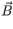# Problem: The earth's magnetic field strength is 5.0x10-5 T. How fast would you have to drive your car to create a 4.0 V motional emf along your 1.0 m -long radio antenna? Assume that the motion of the antenna is perpendicular to .

###### FREE Expert Solution

Induced emf:

$\overline{){\mathbf{\epsilon }}{\mathbf{=}}{\mathbf{-}}\frac{\mathbf{d}\mathbf{\varphi }}{\mathbf{d}\mathbf{t}}}$

Magnetic flux:

θ = 0°

Φ = BA

91% (496 ratings)###### Problem Details

The earth's magnetic field strength is 5.0x10-5 T. How fast would you have to drive your car to create a 4.0 V motional emf along your 1.0 m -long radio antenna? Assume that the motion of the antenna is perpendicular to.